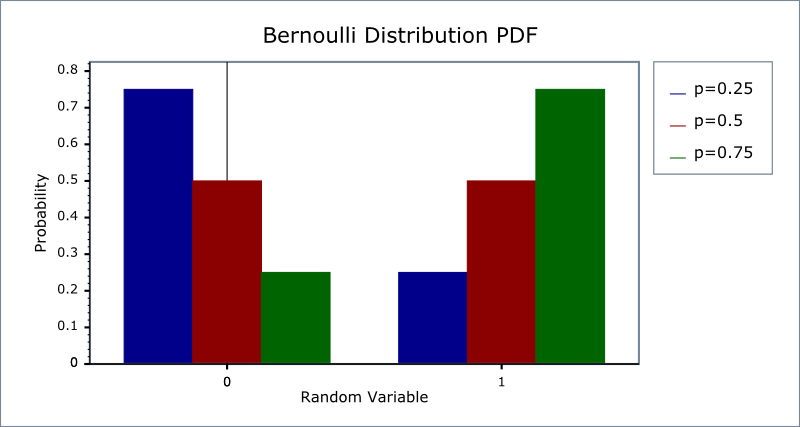Boost C++ Libraries

...one of the most highly regarded and expertly designed C++ library projects in the world.

This is the documentation for an old version of boost. Click here for the latest Boost documentation.
Bernoulli Distribution

#include <boost/math/distributions/bernoulli.hpp>

namespace boost{ namespace math{
template <class RealType = double,
class Policy   = policies::policy<> >
class bernoulli_distribution;

typedef bernoulli_distribution<> bernoulli;

template <class RealType, class Policy>
class bernoulli_distribution
{
public:
typedef RealType  value_type;
typedef Policy    policy_type;

bernoulli_distribution(RealType p); // Constructor.
// Accessor function.
RealType success_fraction() const
// Probability of success (as a fraction).
};
}} // namespaces

The Bernoulli distribution is a discrete distribution of the outcome of a single trial with only two results, 0 (failure) or 1 (success), with a probability of success p.

The Bernoulli distribution is the simplest building block on which other discrete distributions of sequences of independent Bernoulli trials can be based.

The Bernoulli is the binomial distribution (k = 1, p) with only one trial.

probability density function pdf f(0) = 1 - p, f(1) = p. Cumulative distribution function D(k) = if (k == 0) 1 - p else 1.

The following graph illustrates how the probability density function pdf varies with the outcome of the single trial:Member Functions
bernoulli_distribution(RealType p);

Constructs a bernoulli distribution with success_fraction p.

RealType success_fraction() const

Returns the success_fraction parameter of this distribution.

Non-member Accessors

All the usual non-member accessor functions that are generic to all distributions are supported: Cumulative Distribution Function, Probability Density Function, Quantile, Hazard Function, Cumulative Hazard Function, mean, median, mode, variance, standard deviation, skewness, kurtosis, kurtosis_excess, range and support.

The domain of the random variable is 0 and 1, and the useful supported range is only 0 or 1.

Outside this range, functions are undefined, or may throw domain_error exception and make an error message available.

Accuracy

The Bernoulli distribution is implemented with simple arithmetic operators and so should have errors within an epsilon or two.

Implementation

In the following table p is the probability of success and q = 1-p. k is the random variate, either 0 or 1.

NoteThe Bernoulli distribution is implemented here as a strict discrete distribution. If a generalised version, allowing k to be any real, is required then the binomial distribution with a single trial should be used, for example: binomial_distribution(1, 0.25)

Function

Implementation Notes

Supported range

{0, 1}

pdf

Using the relation: pdf = 1 - p for k = 0, else p

cdf

Using the relation: cdf = 1 - p for k = 0, else 1

cdf complement

q = 1 - p

quantile

if x <= (1-p) 0 else 1

quantile from the complement

if x <= (1-p) 1 else 0

mean

p

variance

p * (1 - p)

mode

if (p < 0.5) 0 else 1

skewness

(1 - 2 * p) / sqrt(p * q)

kurtosis

6 * p * p - 6 * p +1/ p * q

kurtosis excess

kurtosis -3